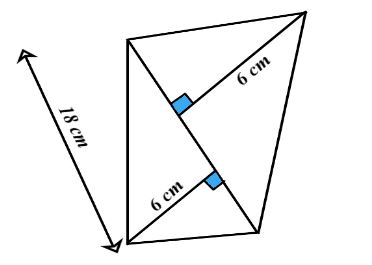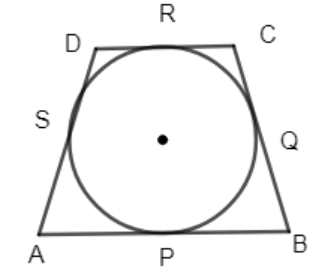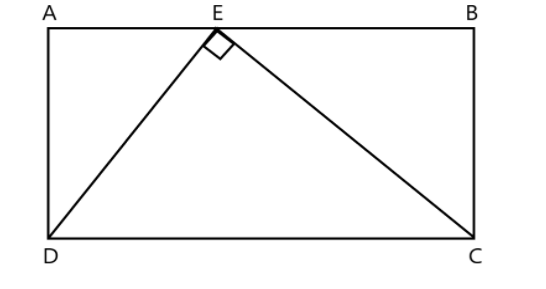Filters
Latest Questions
Mathematics
In the figure, ABCD is a quadrilateral. Name the pairs of opposite angles.Mathematics
Show that the sum of all sides of a quadrilateral is greater than the sum of the diagonals.
Mathematics
Three angles of a quadrilateral are equal. The fourth angle measures $120^\circ$. What is the measure of each of the equal angles?
Mathematics
In an isosceles trapezoid $ABCD$, $AB = CD = 5$. The top base $= 8$ and the bottom base $= 14$. What is the area of this trapezoid?
Mathematics
Prove that the sum of 4 angles in a quadrilateral is $360$?
Mathematics
How to find the area of this quadrilateral?Mathematics
A computer system is advertised with a $14 - inch$ monitor. The measurement of the monitor is along the diagonal of the screen. The height of the monitor screen is $9$ inches. What is the width of the monitor screen?
Mathematics
Find the length of the side if the diagonals of the rhombus are 16 and 18?
Mathematics
In the above, a quadrilateral ABCD is drawn to circumscribe a circle, with center O, in such a way that the sides AB, BC, CD and DA touch the circle at the points P, Q, R and S respectively. Prove that AB + CD = BC + DA.Mathematics
Fill in the blanks:
Mathematics
: In the diagram $ABCD$ is a rectangle and point $E$ lies on $AB$. Triangle $DEC$ has $\left| \!{\underline { {DEC} }} \right. = {90^ \circ }$, $DE = 3$ and $EC = 4$. The length of $AD$ isA.2.4
B.2.8
C.1.8
D.3.2

Mathematics